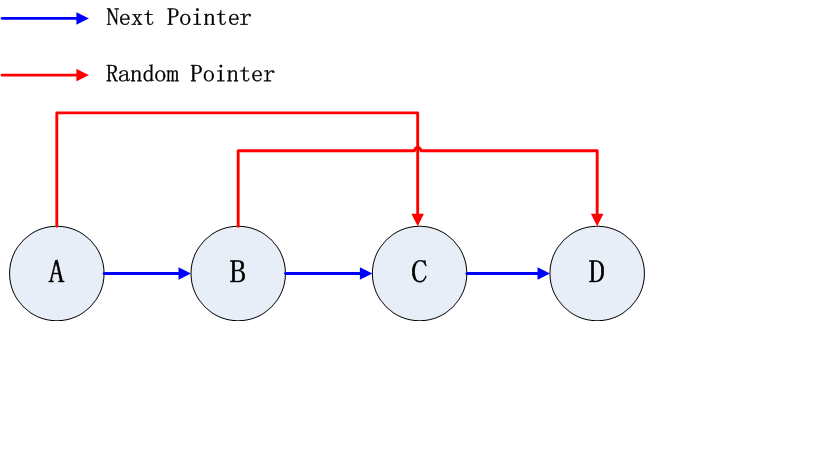Leetcode 138 Copy List with Random Pointer

Leetcode 138 Copy List with Random Pointer

The description of problem is :

```/**
* Definition for singly-linked list with a random pointer.
* class RandomListNode {
*     int label;
*     RandomListNode next, random;
*     RandomListNode(int x) { this.label = x; }
* };
*/

A linked list is given such that each node contains an additional random pointer which could point to any node in the list or null.

Return a deep copy of the list.```

Analysis

This problem seems quite simple at first glance, and so it is. The most straightforward thought is as follow:

```1. Traverse the whole linked list ignoring the random pointer, during which:
- Construct a hashMap between original list nodes with copied list node. Say nodeMap<RandomListNode,RandomListNode>.
- Construct next pointers for copied list

2. Traverse second time the original linked list, during which check the random pointer. Say original node A, copied node A'. Thus we have:

nodeMap.get(A) = A'

3. Now we have:
A'.random = nodeMap.get(A.random)

```

Implementation With HashMapEdit Copy List with Random Pointer on GitHub

Here comes the easiest part of the HashMap implementation:

```/**
* Definition for singly-linked list with a random pointer.
* class RandomListNode {
*     int label;
*     RandomListNode next, random;
*     RandomListNode(int x) { this.label = x; }
* };
*/
public class Solution {
return null;
HashMap<RandomListNode, RandomListNode> map = new HashMap<RandomListNode, RandomListNode>();

p = p.next;
while (p != null) {
RandomListNode temp = new RandomListNode(p.label);
map.put(p, temp);
q.next = temp;
q = temp;
p = p.next;
}

while (p != null) {
if (p.random != null)
q.random = map.get(p.random);
else
q.random = null;

p = p.next;
q = q.next;
}

}
}```

Implementation Without HashMapEdit Copy List with Random Pointer on GitHub

Now think further, what if we can’t use extra space like HashMap or HashTable. What if the only thing we can do is pure linked list manipulation. Well, there is a solution, we just need some copies of the list nodes. Let me do the illustration with following graphs.

Suppose the original list is as follow:Now do the pointer manipulation during first traverse on original list as follow and get the second graph:

```A* = new RandomListNode(A.label);
A*.next = A.next;
A.next = A*;```Now we have copy the random pointer of copied list during second traverse on the merged list:

`A*.random = A.random.next`

At last, we can split the merged list into two during third traverse. Note that we CAN’T do the split and copy random list during the same travers. This is because a node in list can be pointing at previous node in list with random pointer, thus we have to keep the whole structure untouched until all the random pointer have been duplicated.

```A.next = A.next.next;
A*.next = A.next.next;```

Here comes the code:

```/**
* Definition for singly-linked list with a random pointer.
* class RandomListNode {
*     int label;
*     RandomListNode next, random;
*     RandomListNode(int x) { this.label = x; }
* };
*/
public class Solution {
public static RandomListNode copyRandomList(RandomListNode head) {
return null;
}

RandomListNode copyCur = null;

while(cur != null){
copyCur = new RandomListNode(cur.label);
}
copyCur.next = cur.next;
cur.next = copyCur;
cur = cur.next.next;
}

while(cur != null){
cur.next.random = cur.random!=null ? cur.random.next : null;
cur = cur.next.next;
}